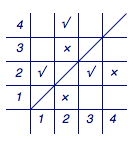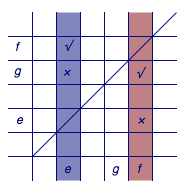# 每日一题 利用极端原理构造

（1）若$n=4$，$\left(a_1,a_2\right)$，$\left(a_3,a_2\right)$，$\left(a_2,a_4\right)\in T$，求$l_T\left(a_2\right)$的值及$l_T\left(a_4\right)$的最大值；

（2）从$l_T\left(a_1\right)$，$l_T\left(a_2\right)$，$\cdots$，$l_T\left(a_n\right)$中任意删去两个数，记剩下的$n-2$个数的和为$M$．求证：$M\geqslant \dfrac 12n(n-5)+3.$

（3）对于满足$l_T\left(a_i\right)<n-1$（$i=1,2,3,\cdots,n$）的每一个集合$T$，集合$S$中是否都存在三个不同的元素$e$，$f$，$g$，使得$d_T\left(e,f\right)+d_T\left(f,g\right)+d_T\left(g,e\right)=3$恒成立，并说明理由．（1）我们引入“坐标”来理解题意．将$\left(a_i,a_j\right)\in T$用坐标$\left(i,j\right)$的位置打“√”表示，相应地，此时$\left(a_j,a_i\right)\not\in T$用坐标$\left(j,i\right)$的位置打“×”表示．这样一来就有任何一个“√”关于$y=x$对称的位置均为“×”，且$l_T\left(a_i\right)$表示第$i$列中的“√”的总数．那么有下图．从而$l_T\left(a_2\right)=1$，且$l_T\left(a_4\right)$最大为$2$； （2）根据题意得$\begin{split}M&\geqslant \sum_{i=1}^n{l_T\left(a_i\right)}-\left(n-1\right)-\left(n-2\right)\\&=\dfrac{1}{2}n\left(n-1\right)-2n+3\\&=\dfrac 12n^2-\dfrac 52n+3,\end{split}$于是原不等式成立． （3）答案是肯定的，这里需要利用极端原理进行构造．如图，设$l_T{\left(a_f\right)}$最大．根据题意$l_T{\left(a_f\right)}\leqslant{n-1}$，于是第$f$列中必然存在一个“×”，设其坐标为$\left(f,e\right)$，于是在$\left(e,f\right)$处为“√”． 逐行比较第$e$列和第$f$列中的数．由于这两列在第$f$行和第$e$行的较量中第$e$列多出一个“√”，因此在其他行的较量中必然存在某一行为第$e$列为“×”而第$f$列为“√”的情况（否则与$l_T{\left(a_f\right)}$最大矛盾），设该行为第$g$行． 这样一来，我们就有坐标$\left(e,f\right)$，$\left(f,g\right)$，$\left(g,e\right)$处均为“√”，命题得证．

### 2 Responses to 每日一题 利用极端原理构造

1. Pingback引用通告： 每日一题保三角形函数 | 数海拾贝内容系统

2. Pingback引用通告： 每日一题保三角形函数 | Math173